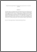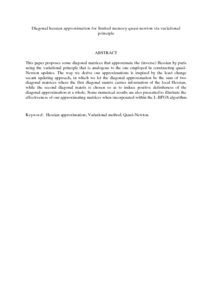# Diagonal hessian approximation for limited memory quasi-newton via variational principle

## Citation

Marjugi, Siti Mahani and Leong, Wah June (2013) Diagonal hessian approximation for limited memory quasi-newton via variational principle. Journal of Applied Mathematics, 2013. art. no. 523476. pp. 1-8. ISSN 1110-757X; ESSN: 1687-0042

## Abstract

This paper proposes some diagonal matrices that approximate the (inverse) Hessian by parts using the variational principle that is analogous to the one employed in constructing quasi-Newton updates. The way we derive our approximations is inspired by the least change secant updating approach, in which we let the diagonal approximation be the sum of two diagonal matrices where the first diagonal matrix carries information of the local Hessian, while the second diagonal matrix is chosen so as to induce positive definiteness of the diagonal approximation at a whole. Some numerical results are also presented to illustrate the effectiveness of our approximating matrices when incorporated within the L-BFGS algorithm.Preview
PDF (Abstract)
Diagonal hessian approximation for limited memory quasi.pdfView Item